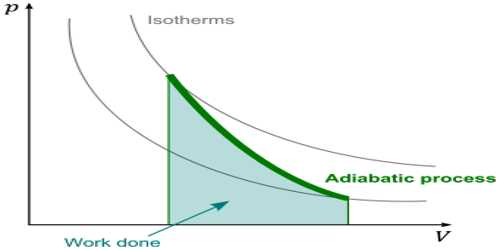# Use of First Law of Thermodynamics in Adiabatic Process

Use of First Law of Thermodynamics in adiabatic process

The process in which heat in a system remains constant but pressure and the volume change is called adiabatic process. In thermodynamics, an adiabatic process is one that occurs without transfer of heat or matter between a thermodynamic system and its surroundings. In an adiabatic process, energy is transferred to its surroundings only as work. In an adiabatic process, heat is not exchanged. So, in case of adiabatic expansion of a gas, dQ = 0

The first law of thermodynamics can be written as, dQ = du + dW …. ….. (1)

From equation (1) we get, dQ = O = du + dW

or, du = – dW … …. (2)

If the initial internal energy of a gas is u1 and the final internal energy is u2, then equation (2) can be written as, du = u2 – u1 = – dW …. …. (3)

so, u2 < u1 … … (4)

That means, during adiabatic expansion internal energy of the system decreases due to external work is done, consequently, temperature also decreases.

Similarly, also in case of adiabatic contraction or conservation dQ = 0. In case of contraction work is done on the system, so W is negative. Hence from equation (3) we get.

du = – (- dw) = dw … ….. (5)

or, u2 – u1 = dW, where u1 and u2 are respectively the initial and final internal energies.

so, u2 > u1 … … (6)

That means, during adiabatic contraction, the internal energy of the gas increases, so the temperature of the gas increases. Equations (4) and (6) are the mathematical form of the first law of thermodynamics in the adiabatic process. An example of an adiabatic process is a gas expanding so quickly that no heat can be transferred. The expansion does work, and the temperature drops. This is exactly what happens with a carbon dioxide fire extinguisher, with the gas coming out at high pressure and cooling as it expands at atmospheric pressure.

Example: During adiabatic expansion, the internal energy of the system decreases. But why does temperature increase during adiabatic contraction?

During adiabatic expansion won dune by the system is performed by the internal energy of the system and hence the internal energy of the system decreases. That means the system becomes cool. On the other hand, during adiabatic contraction work is done on the system by the energy supplied from outside. Hence internal energy is increased. As a result, the temperature of the system increases as well.